# ncert maths solutions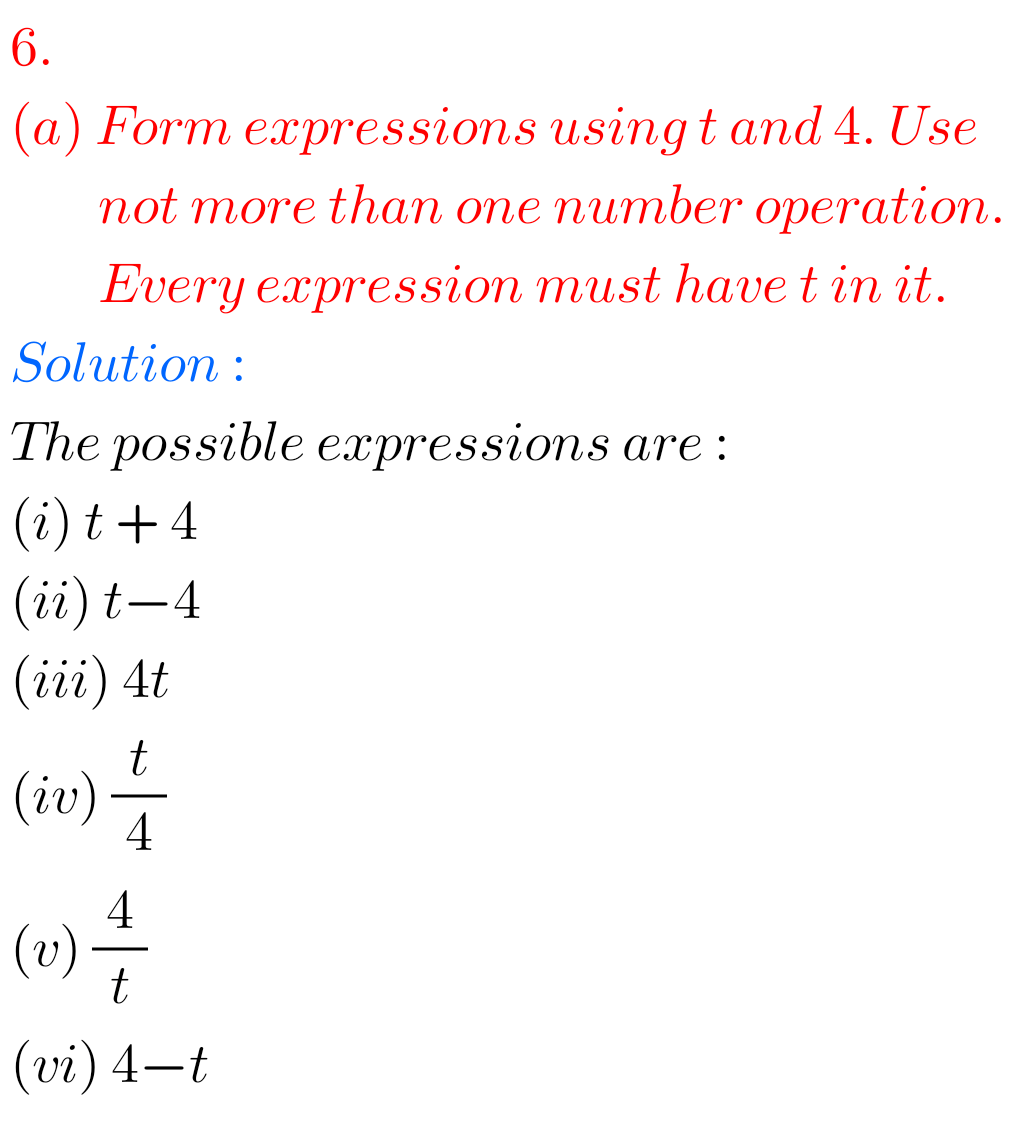## Algebra solutions for exercise 11.3 class 6 Ncert Maths

Solutions for Algebra Exercise 11.3 Chapter 11 class 6 Ncert Maths Class 6 chapter 11 Algebra exercise 11.3 solutions  Maths Ncert textbook are given. Study the textbook lesson Algebra very well. Observe the example problems and solutions given in the solutions. Observe the solutions and try them in your own method. You can also see …

## Chapter 11 Algebra exercise 11.2 solutions Class 6  Ncert Maths

Algebra chapter 11 exercise 11.2 solutions Ncert Maths Class 6 chapter 11 Algebra exercise 11.2 solutions  Maths Ncert textbook are given. Study the textbook lesson Algebra very well  Observe the example problems and solutions given in the solutions. Observe the solutions and try them in your own method. You can also see Ncert Maths Solutions …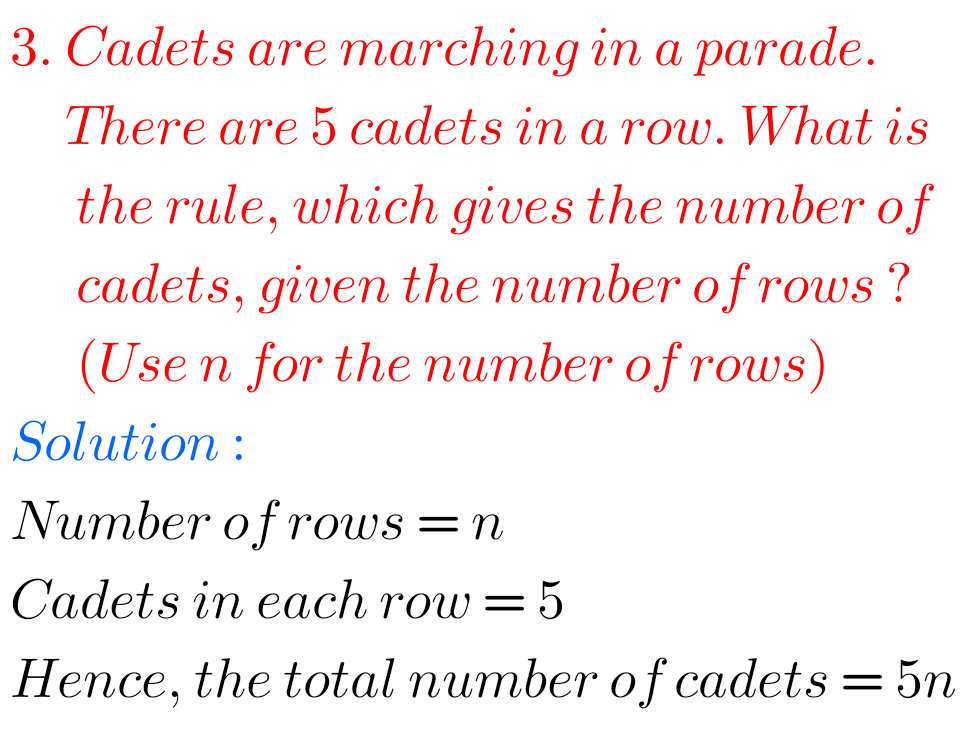## Ncert solutions for maths class 6 Algebra exercise 11.1 chapter 11

Maths class 6 chapter 11 exercise 11.1 Ncert solutions Class 6 chapter 11 Algebra exercise 11.1 solutions  Maths Ncert textbook are given. Study the textbook lesson Algebra very well Observe the example problems and solutions given in the solutions. Observe the solutions and try them in your own method. You can also see Ncert Maths …## Ncert solutions class 6 Maths Decimals chapter 8 exercise 8.2

Decimals Solutions class 6 maths exercise 8.2 Ncert Maths Ncert Class 6 textbook Chapter 8 Decimals Exercise 8.2 solutions are given. Study the textbook lesson Decimals very well. Observe the example problems and solutions given in the textbook. Observe the given solutions and try them in your own method. You can see Ncert maths solutions …## Ncert solutions for Mensuration chapter 10 exercise 10.3 class 6

Class 6 chapter 10 Mensuration exercise 10.3 solutions Class 6 Maths Ncert Chapter 10 Mensuration Exercise 10.3 solutions are given. Study the tectbook lesson Mensiration very well. Observe the example problems and solutions given in the tectbook. Observe the given solutions and try them in your own method. You can see Ncert maths solutions for …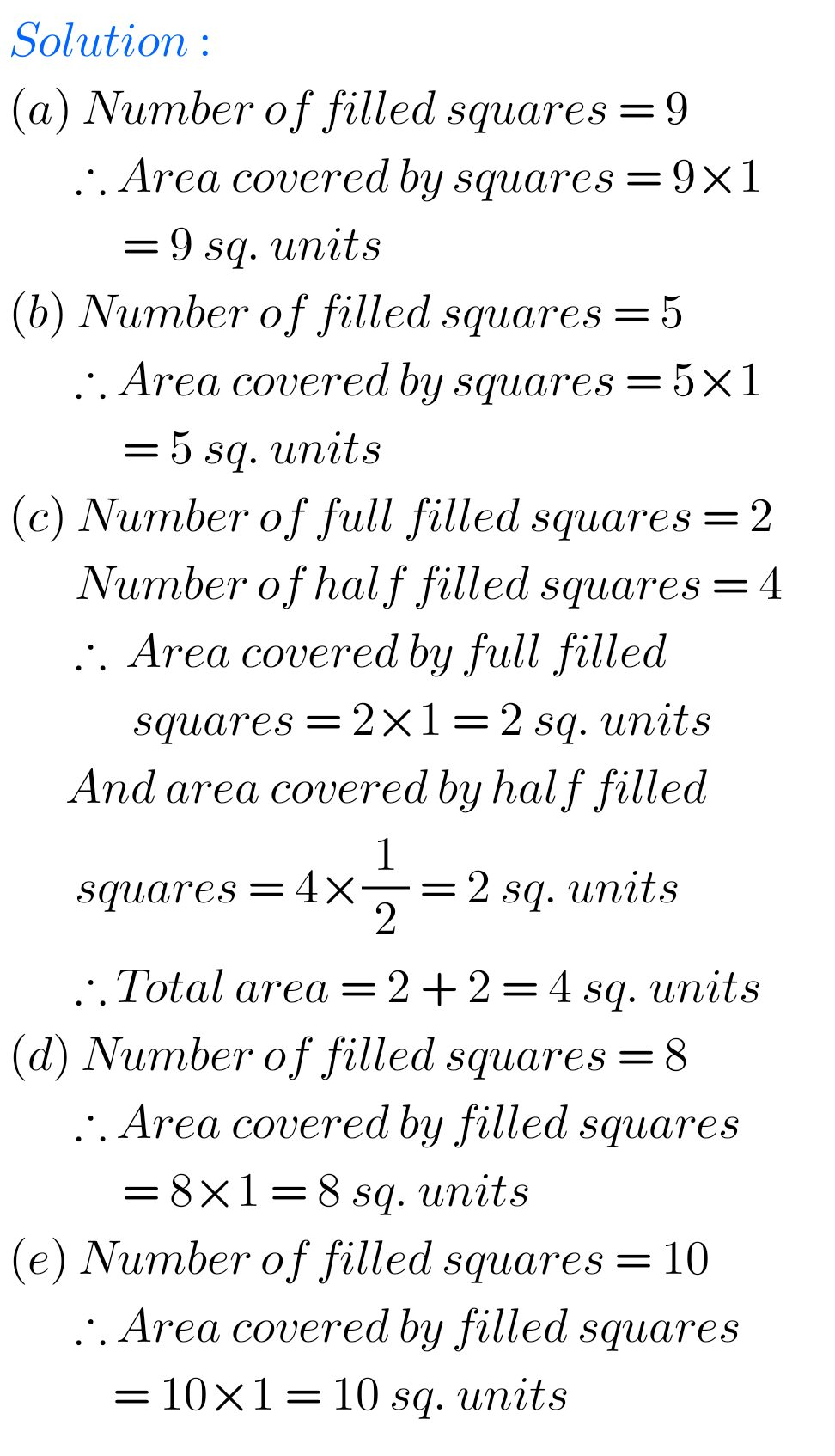## Mensuration Exercise 10.2 solutions chapter 10 class 6 ncert maths

Ncert solutions class 6 maths exercise 10.2 Mensuration Class 6 Maths Ncert Chapter 10 Mensuration Exercise 10.2 solutions are given. Study the tectbook lesson Mensiration very well. Observe the example problems and solutions given in the tectbook. Observe the given solutions and try them in your own method. You can see Ncert maths solutions for …## Ncert Maths solutions for Mensuration chapter 10 exercise 10.1 class 6

Mensuration solutions class 6 maths exercise 10.1 Class 6 Maths Ncert Chapter 10 Mensuration Exercise 10.1 solutions are given. Study the tectbook lesson Mensiration very well. Observe the example problems and solutions given in the tectbook. Observe the given solutions and try them in your own method. You can see Ncert maths solutions for class …## Class 6 Decimals Chapter 8 exercise 8.6 solutions Maths Ncert

6th class maths Decimals exercise 8.6 solutions Ncert Maths Ncert Class 6 textbook Chapter 8 Decimals Exercise 8.6 solutions are given. Study the textbook lesson Decimals very well. Observe the example problems and solutions given in the textbook. Observe the given solutions and try them in your own method. You can see Ncert maths solutions …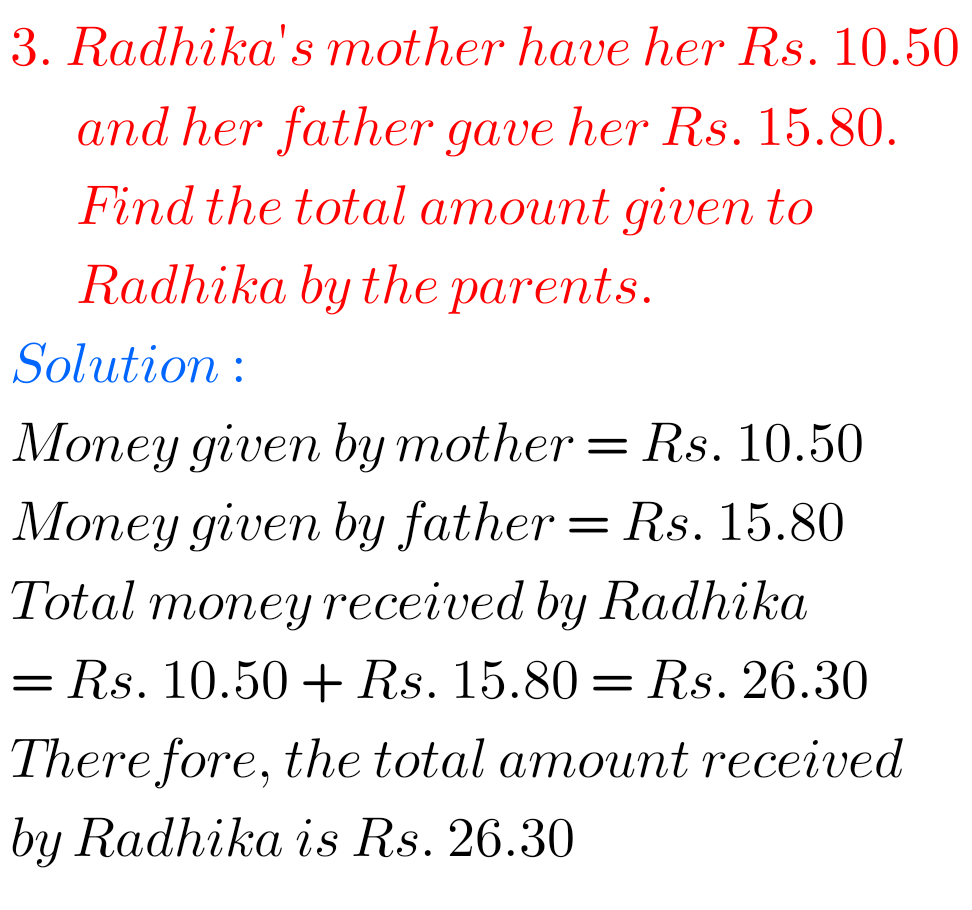## Exercise 8.5 Decimals chapter 8 solutions class 6 Maths Ncert

Decimals chapter 8 exercise 8.5 solutions Ncert maths class 6 Maths Ncert Class 6 textbook Chapter 8 Decimals Exercise 8.5 solutions are given. Study the textbook lesson Decimals very well. Observe the example problems and solutions given in the textbook. Observe the given solutions and try them in your own method. You can see Ncert …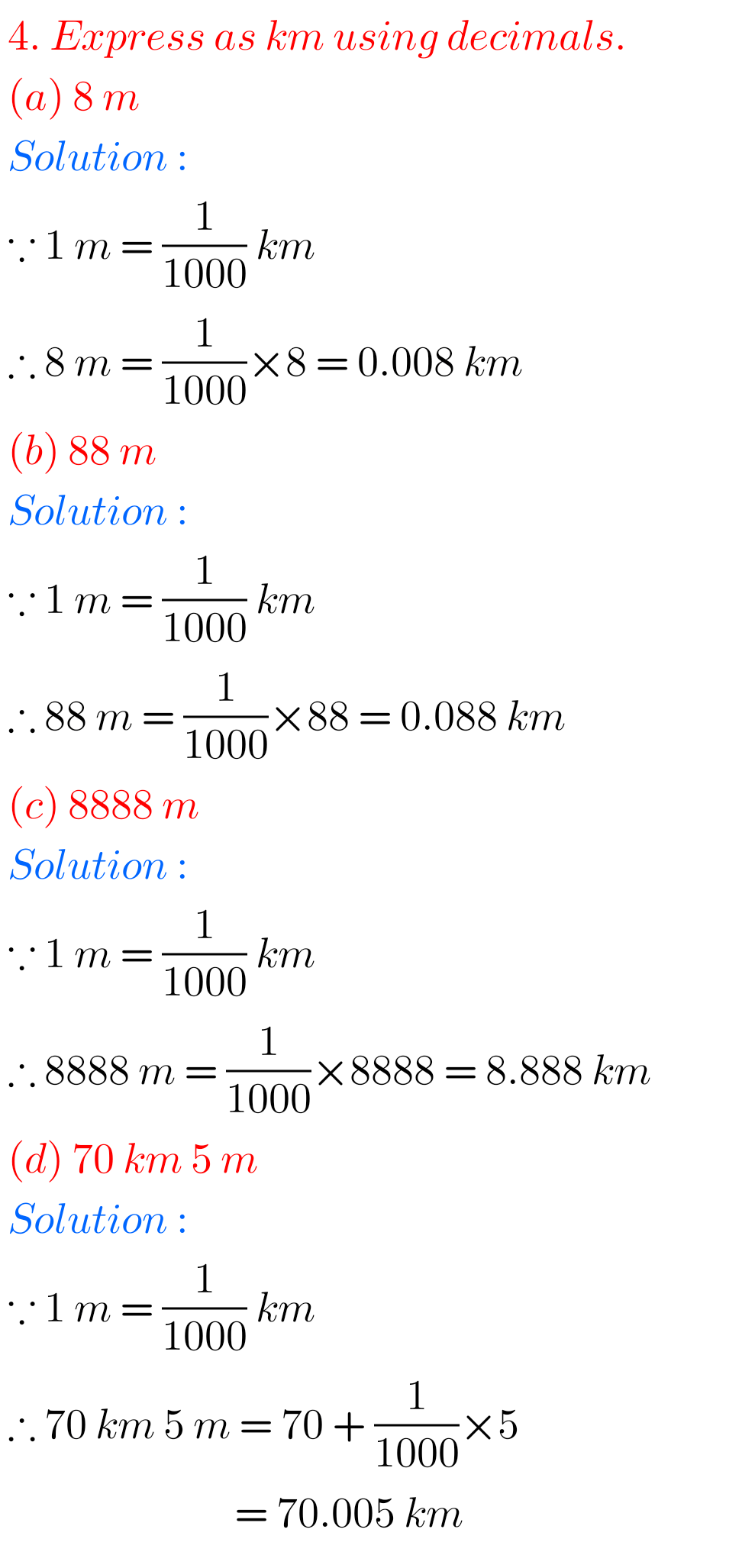## Chapter 8 exercise 8.4 Decimals solutions class 6 maths Ncert

Class 6 Exercise 8.4 solutions Decimals Ncert Maths Maths Ncert Class 6 textbook Chapter 8 Decimals Exercise 8.1 solutions are given. Study the textbook lesson Decimals very well. Observe the example problems and solutions given in the textbook. Observe the given solutions and try them in your own method. You can see Ncert maths solutions …